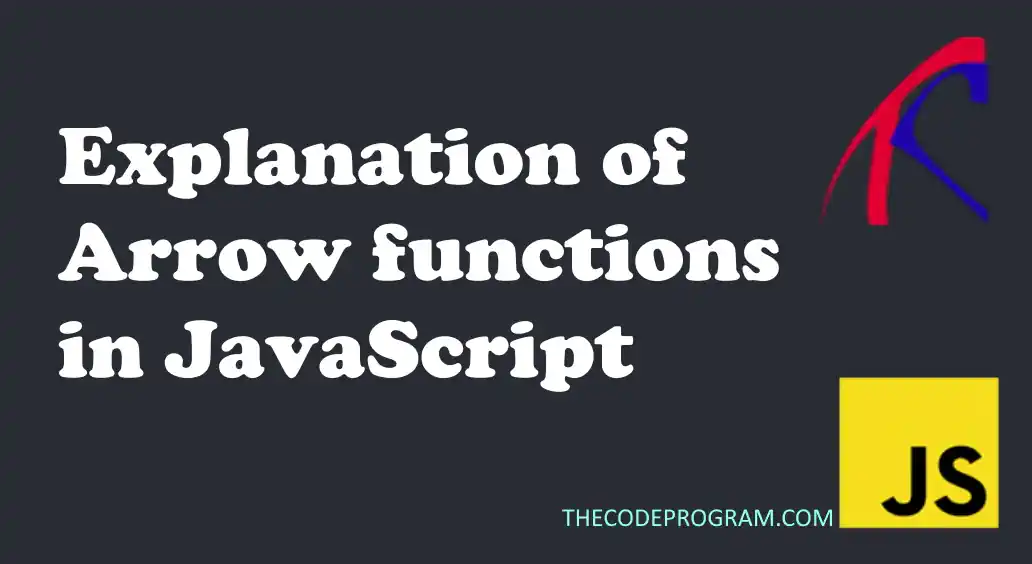## Explanation of Arrow functions in JavaScript

Hello everyone, in this article we are going to talk about how to use arrow functions in Javascript with the helps of examples for better understanding.

Let's get started

## What is Arrow Function?

Arrow function is introduced to create functions as cleaner for better understanding the functions. They introduced with the ES6 standarts to JavaScript. With Arrow functions we can create functions with no additional parenthesis and we can declare some inline functions. We can increase the flexibility. We can declare functions inside functions, We can declare functions inside some data types and invoke them

Firstly let's take a look at how to declare an arrow function. There is a couple of types arrow function declarations. Firstly let's take a look at them.

• If we have single line code for the function:
``````
const func = () => console.log("Thecodeprogram");
``````
• If we have single line code for the function with parameter:
``````
const print = (text) => console.log(text);
``````
• If we have multiple line code for the function:
``````
const myFunction = (arguments,...) => {
//method body
}
``````
• If we need ternary operator with a condition
``````
const myFunction = (booleanCondition) => booleanCondition ? { /*method body*/ } : { /*method body*/ }
``````

##### Example 1:
``````
const calculatePayment = (salary, taxPercentage) => salary - (salary * taxPercentage /100);
console.log("Salary of Burak is : "+ calculatePayment(15000, 18));
console.log("Salary of Hamdi is : "+ calculatePayment(18000, 25));
``````

In this example we declared a single line function with two parameters and we printed the result. Below you can see the example output:

``````
Salary of Burak is : 12300
Salary of Hamdi is : 13500
``````

##### Example 2:
``````
const user = {
"name":"Burak Hamdi",
"surname":"TUFAN",
"salary":8000,
"year":2015
}

const showUserDetail = (data) => {
let result = "Name : " + data.name + "\n";
result += "Surname : " + data.surname + "\n";
result += "Total Paid Salary : " + ((new Date().getFullYear() - data.year) * data.salary) + "\n";
return result;
}
console.log(showUserDetail(user));
``````
Program output will be like below
``````
Name : Burak Hamdi
Surname : TUFAN
Total Paid Salary : 56000
``````

In this example we have created an arrow function to create details from a JSON data. As you can see this process is not a single line code, so we declared an arrow function with multi line and at the end returned the extracted value from JSON data.

##### Example 3:
In this example lets declare an arrow function inside another function.
``````
const emp1 = {
"name":"The",
"year":2008,
"level":4
}
const emp2 = {
"name":"Code",
"year":2015,
"level":2
}
const emp3 = {
"name":"Program",
"year":2002,
"level":8
}

const showLevelIncreasings = (...employees) => {
let result = "";
const levelIncrease = (emp) => (new Date().getFullYear() - emp.year) > (emp.level * 3) ? "Can increase" : "Can not increase";
for(let i=0; i<employees.length; i++){
result += employees[i].name + "\n";
result += "Level increase status : " + levelIncrease(employees[i]) + "\n";
result += "---------------------" + "\n";
}
return result + "\n" + "\n";
}

console.log(showLevelIncreasings(emp1,emp2,emp3));
``````
Program output will be like below
``````
The
Level increase status : Can increase
---------------------
Code
Level increase status : Can increase
---------------------
Program
Level increase status : Can not increase
``````

In this example we have declared a method to extract some specfic datas. In this method we also declared an arrow function. We just return a value in this ternary function, but if we want to make more complicated logics inside we can also increase the code of return parts.

##### Example 3:
``````
const nameArr = ["burak", "hamdi", "tufan","the", "code", "program"];
const printUpperCase = (text) => console.log(text.toUpperCase() + "\n");
nameArr.forEach((name) => printUpperCase(name));
``````
Program output will be like below
``````
BURAK
HAMDI
TUFAN
THE
CODE
PROGRAM
``````

As you can see we declared an arrow function to manage the texts and we directly called it from our foreach function and we printed all of them with upper case. We did not need to declare another function inside the forEach and we directly called our new function.

As you can see we used arrow functions in variaous ways to calculate some datas in more flexible ways. With arrow functions we can declared processes with cleaner and understandable ways.

Burak Hamdi TUFAN

18/05/2020

16/11/2021

23/10/2022